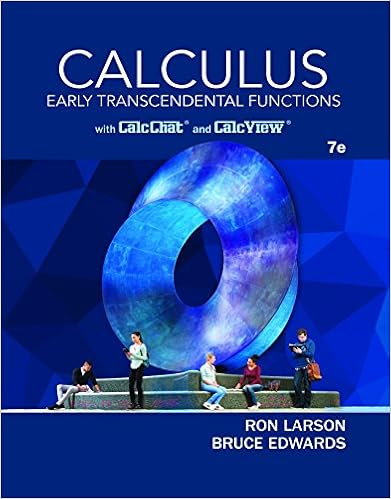# Solution solution continued exercise 11 8 points

• Test Prep
• 26

This preview shows page 21 - 26 out of 26 pages.

##### We have textbook solutions for you!
The document you are viewing contains questions related to this textbook.The document you are viewing contains questions related to this textbook.
Chapter 14 / Exercise 1
Calculus: Early Transcendental Functions
Edwards/LarsonExpert Verified
Solution:
##### We have textbook solutions for you!
The document you are viewing contains questions related to this textbook.The document you are viewing contains questions related to this textbook.
Chapter 14 / Exercise 1
Calculus: Early Transcendental Functions
Edwards/LarsonExpert Verified
Solution (continued):
Exercise 11 (8 points):Compute the determinant of the following matrix (show yourwork):A=1000012222103001034410305Solution:
Solution (continued):
Exercise 12 (12 points):Consider the following matrix:A=0111101111011110(1) Write down the characteristic polynomialfA(X). What are the real eigenvaluesofA, and their corresponding algebraic multiplicities?(2)Ais diagonalizable. Find a basis ofR4consisting of eigenvectors ofA.(3) Find an orthonormal basis ofR4consisting of eigenvectors ofA.(4) Use your answer to computeA7by diagonalizingA.Solution:
Solution (continued):
•••